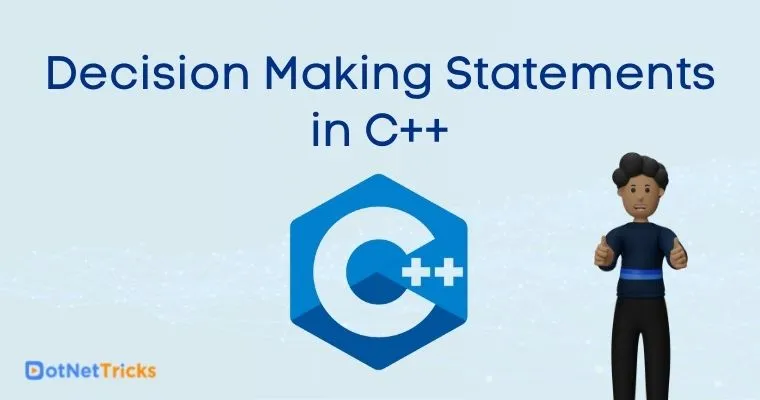# Decision Making Statements in C++

22 Aug 2022
Intermediate
36.6K Views

Welcome readers. If you are here directly, it is assumed that you know the basics of C++ and the types of data types, variables and operators in C++ programming language. If you are new to this, we recommend you refer the Getting Started with C++ Language article and the articles on data types, variables and operators in C++. Let’s get started with the different types of decision-making statements in C++.

## What are Decision Making Statements

Decision making statements allow you to decide the order of execution of specific statements in your program. You can set up a condition and tell the compiler to take a particular action if the condition is met. In case the condition is not met, you can instruct the compiler to execute a different block of code. The decision-making statements available in C++ are :

• if statement

• if .. else statement

• nested if statements

• switch statement

### if statement

If statement is the basic decision-making statement that tells the compiler to execute a code block only if the condition is true. For example, if the students’ marks are above 40, print their results as pass. Otherwise, print the result as fail. The syntax of if statement will be :

```if (condition)
{
// execute statement 1;
// execute statement 2;
…..
}
```
Example Program
```#include <iostream>
using namespace std;

int main()
{
int a;
cout << "Enter a number" << '\n';
cin >> a;
if (a >10)
cout<<"The entered number is greater than 10";
}
```

If the input is 5, the output of this program will be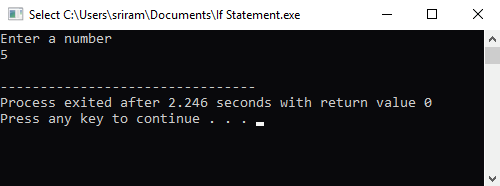If the input is 20, the output of this program will be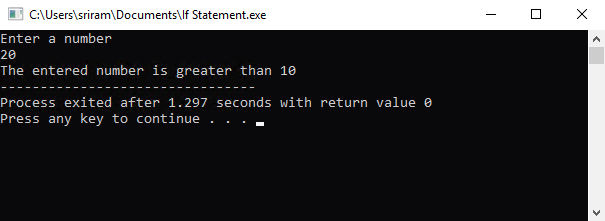### If .. Else Statement

The if .. else statement works in the same way as the if statement. The only difference is that you can define what the compiler should do if the condition is false. Considering the same example as the if statement, you can tell the compiler to print a message as “The entered number is less than 10”. The syntax of if .. else statement will be :

```if (condition)
{
// execute statement 1;
// execute statement 2;
…..
}
else
{
// execute statement 3;
// execute statement 4;
…..
}
```
Example Program
```#include <iostream>
using namespace std;

int main()
{
int a;
cout << "Enter a number" << '\n';
cin >> a;
if (a > 10)
cout <<"The entered number is greater than 10";
else
cout <<"The entered number is less than 10";
}
```

If the input is 5, the output of this program will be :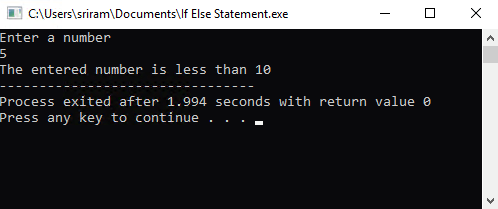If the input is 20, the output of this program will be :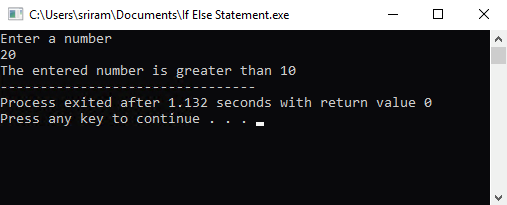### Nested if statements

Nested if statements means “One if statement within another if statement”. There may be situations where you may need to evaluate a condition based on the result of another condition. In these cases, nested if statements will come in handy.The syntax of nested if statement will be :

```if (condition 1)
{
if (condition 2)
{
if (condition 3)
{
// execute statement 1;
}
if (condition 4)
{
// execute statement 2;
}
}
}
```
Example Program
``` #include <iostream>
using namespace std;

int main()
{
int a;
cout << "Enter a number" << '\n';
cin >> a;
if(a>=10)
{
if(a>=20)
{
if(a>=100)
{
cout<<"You entered a value greater than 100." << '\n';
}
if(a<=75)
{
cout<<"You entered a value less than 75" << '\n';
}
}
if(a<=30)
{
cout<<"You entered a value less than 30" << '\n';
}
}
if(a<10)
{
cout<<"You entered a value less than 10" << '\n';
}
}
```

If the input is 2, the output of this program will be :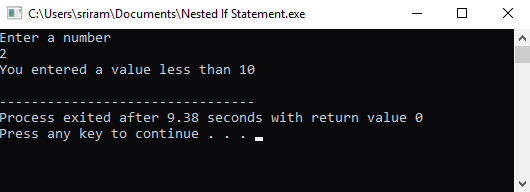If the input is 150, the output of this program will be :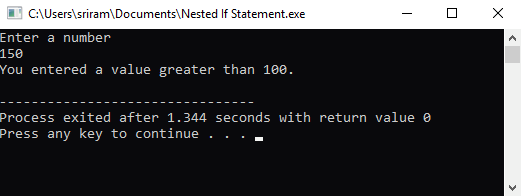### If – else – if Ladder

In this decision-making statement, the compiler will execute the statement from the top to bottom. The compiler will look for the condition to match true. Until the positive result is reached, the compiler will traverse through the if-else-if loop. Once the condition matches to true, it will execute the piece of code within the success loop. If none of the conditions are true, the final else statement will get executed. If the final else statement is not available, no action will take place. The syntax of if – else – if ladder will be :

```if (condition 1)
statement;
else if (condition 2)
statement;
else if (condition 3)
statement;
…
…
else
statement;
// execute statement 2;
}
```
Example Program
``` #include <iostream>
using namespace std;

int main()
{
int a, b, c;
cout << "Enter any two numbers : " << '\n';
cin >> a >> b;
if (a > b)
{
cout << "The value of a is greater than b" << '\n';
}
else if (a < b)
{
cout << "The value of b is greater than a" << '\n';
}
else
{
cout << "You have entered equal values" << '\n';
}
}
```

The output of this program will be :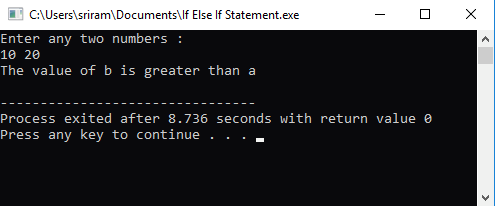### Switch Statement

You can use the switch statement when you want to evaluate a condition against different cases (expressions). Depending on the input, the appropriate case will be executed, and the result will be printed. The syntax of switch case statement will be :

```switch (condition)
{
case expression1:
//statement to execute;
break;
case expression2:
//statement to execute;
break;
case expression3:
//statement to execute;
break;
…
default:
// statement to execute;
}
```
Example Program
``` #include <iostream>
using namespace std;

int main()
{
int a;
cout << "Enter a number between 1 to 7 : " << '\n';
cin >> a;
switch (a)
{
case 1:
{
cout << "The first colour of the rainbow is VIOLET: " << '\n';
break;
}
case 2:
{
cout << "The second colour of the rainbow is INDIGO: " << '\n';
break;
}
case 3:
{
cout << "The third colour of the rainbow is BLUE: " << '\n';
break;
}
case 4:
{
cout << "The fourth colour of the rainbow is GREEN: " << '\n';
break;
}
case 5:
{
cout << "The fifth colour of the rainbow is YELLOW: " << '\n';
break;
}
case 6:
{
cout << "The sixth colour of the rainbow is ORANGE: " << '\n';
break;
}
case 7:
{
cout << "The seventh colour of the rainbow is RED: " << '\n';
break;
}
default:
cout << "You have entered a number other than 1 - 7. Please try again!" << '\n';
}
}
```

The output of this program will be :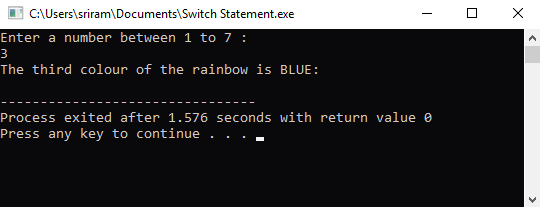## Points to remember

1. Only when a break statement is specified, the compiler will exit from the switch case to the next line after the switch statement.

2. It is not mandatory to specify a break statement after each switch statement. When there is no break, the flow will continue till you specify a break statement.

3. The default case at the end of the switch case will execute when none of the switch case is true.

4. The switch statement cannot handle range values. The switch case label should be a single value.

5. You cannot specify a floating point value for the switch statement. Only integer and character data types are allowed.

6. You can use switch statement when you want to check if an entered value matches the specific case (equality check). If you want to evaluate a logical expression or a relational expression, you can use the If .. else statement.

##### Summary

In this section, we have taken a look at the different decision-making statements in C++ programming language.

Learn to Crack Your Technical Interview